### Jimi HendrixHover over the thumbnail for a full-size version.

Author im_bad_at_N 2009 author:im_bad_at_n avatarnart dronie-nom ibannart nart rated 2009-09-27 2010-07-25 4 by 63 people. \$Jimi Hendrix#im_bad_at_N#dronie-nom#00000000000000000000000000000000000000000000000000000000000000000000000000000000000000000000000000000000000000000000000000000000000000000000000000000000000000000000000000000000000000000000000000000000000000000000000000000000000000000000000000000000000000000000000000000000000000000000000000000000000000000000000000000000000000000000000000000000000000000000000000000000000000000000000000000000000000000000000000000000000000000000000000000000000000000000000000000000000000000000000000000000000000000000000000000000000000000000000000000000000000000000000000000000000000000000000000000000000000000000000000000000000000000000000000000000000000000000000000000000000000000000000000000000000000000000000000000000000000000|12^576,576!12^576,564!12^576,570!12^564,576!12^570,576!12^570,570!12^564,570!12^582,570!12^582,576!12^588,576!12^588,570!12^594,570!12^594,570!12^594,570!12^594,576!12^600,576!12^618,576!12^612,576!12^606,576!12^600,570!12^612,570!12^606,570!12^618,570!12^612,564!12^618,564!12^612,558!12^600,558!12^600,564!12^606,564!12^606,558!12^606,552!12^600,552!12^594,552!12^594,558!12^594,564!12^588,564!12^582,558!12^582,564!12^588,558!12^588,552!12^582,552!12^576,552!12^576,558!12^570,564!12^570,558!12^570,552!12^570,546!12^570,540!12^570,534!12^570,528!12^570,522!12^570,510!12^570,498!12^570,486!12^570,474!12^570,456!12^570,450!12^570,444!12^570,468!12^570,462!12^570,480!12^570,492!12^570,504!12^570,516!12^576,474!12^582,474!12^582,480!12^582,492!12^588,504!12^582,516!12^582,522!12^588,534!12^594,534!12^594,540!12^594,546!12^582,546!12^576,546!12^576,540!12^582,540!12^588,540!12^588,546!12^576,534!12^582,534!12^582,528!12^576,528!12^576,522!12^576,516!12^576,516!12^576,510!12^576,504!12^576,498!12^576,492!12^576,492!12^576,486!12^576,480!12^582,486!12^588,492!12^588,498!12^582,498!12^582,504!12^582,510!12^588,510!12^588,516!12^588,522!12^588,528!12^594,528!12^594,516!12^594,510!12^594,504!12^594,498!12^600,492!12^594,486!12^594,492!12^594,522!12^600,522!12^606,510!12^606,504!12^606,498!12^600,498!12^600,510!12^600,510!12^600,516!12^600,504!12^606,516!12^606,522!12^606,534!12^606,534!12^606,540!12^606,546!12^600,546!12^600,540!12^600,528!12^600,534!12^606,528!12^588,486!12^588,480!12^588,474!12^588,468!12^588,462!12^588,462!12^588,456!12^588,450!12^588,438!12^588,432!12^588,432!12^588,420!12^588,414!12^588,408!12^588,402!12^588,390!12^588,384!12^588,378!12^588,372!12^588,366!12^588,360!12^588,354!12^588,354!12^594,354!12^594,360!12^594,366!12^594,378!12^594,390!12^594,402!12^594,414!12^594,420!12^594,432!12^594,438!12^594,444!12^594,456!12^594,462!12^594,468!12^594,474!12^594,480!12^594,450!12^582,438!12^588,444!12^582,444!12^582,450!12^582,456!12^582,462!12^582,468!12^576,468!12^576,462!12^576,456!12^576,450!12^576,444!12^576,438!12^576,426!12^576,420!12^576,408!12^576,402!12^582,408!12^582,408!12^582,426!12^582,432!12^582,414!12^582,420!12^576,414!12^576,432!12^558,444!12^564,438!12^564,438!12^570,438!12^564,432!12^564,414!12^564,408!12^570,408!12^570,414!12^570,426!12^570,432!12^570,420!12^564,420!12^564,426!12^564,564!12^564,558!12^564,552!12^564,546!12^564,540!12^564,534!12^564,528!12^564,516!12^564,510!12^564,504!12^564,492!12^564,486!12^564,480!12^564,474!12^564,468!12^564,456!12^564,450!12^564,444!12^564,462!12^564,498!12^564,522!12^606,492!12^612,492!12^612,492!12^618,492!12^624,492!12^624,492!12^630,492!12^612,510!12^612,504!12^618,510!12^612,516!12^612,522!12^612,528!12^612,540!12^612,546!12^612,534!12^600,480!12^600,486!12^606,486!12^612,486!12^618,486!12^624,486!12^618,480!12^618,480!12^606,480!12^606,480!12^612,480!12^612,474!12^606,474!12^600,474!12^600,468!12^606,468!12^606,462!12^600,462!12^600,456!12^606,456!12^606,444!12^600,450!12^606,450!12^600,438!12^600,438!12^606,426!12^600,444!12^606,438!12^606,432!12^600,432!12^600,426!12^594,426!12^588,426!12^552,564!12^558,564!12^558,558!12^558,558!12^558,546!12^558,540!12^558,522!12^558,516!12^558,504!12^558,498!12^558,492!12^558,480!12^558,474!12^558,468!12^558,456!12^558,450!12^558,462!12^558,486!12^558,504!12^558,510!12^558,528!12^558,534!12^558,552!12^552,558!12^552,546!12^552,534!12^552,522!12^552,510!12^552,504!12^552,498!12^552,486!12^552,486!12^552,480!12^552,468!12^552,456!12^552,450!12^552,444!12^552,462!12^552,474!12^552,492!12^552,516!12^558,528!12^552,528!12^552,540!12^552,552!12^558,438!12^552,438!12^558,432!12^552,432!12^552,426!12^558,426!12^558,414!12^552,414!12^552,420!12^558,420!12^558,408!12^552,408!12^522,438!12^522,444!12^522,450!12^528,450!12^528,444!12^534,444!12^534,450!12^546,450!12^540,444!12^546,444!12^540,450!12^546,438!12^540,438!12^540,432!12^546,432!12^546,426!12^546,420!12^546,414!12^546,408!12^540,408!12^534,414!12^540,420!12^540,426!12^540,414!12^534,420!12^528,456!12^528,462!12^522,468!12^522,480!12^522,492!12^528,504!12^528,510!12^534,522!12^534,534!12^540,540!12^540,540!12^546,546!12^546,540!12^546,534!12^546,528!12^546,522!12^546,516!12^546,510!12^546,504!12^546,498!12^546,492!12^546,486!12^546,480!12^546,474!12^546,474!12^546,468!12^546,468!12^546,462!12^546,456!12^540,456!12^534,456!12^534,462!12^540,462!12^540,474!12^540,486!12^540,492!12^540,498!12^540,510!12^540,516!12^540,522!12^540,528!12^540,534!12^540,504!12^540,480!12^540,468!12^534,468!12^528,468!12^528,474!12^534,480!12^534,480!12^534,474!12^528,480!12^528,492!12^528,498!12^528,486!12^534,486!12^534,492!12^534,504!12^534,510!12^534,516!12^534,498!12^522,486!12^522,474!12^522,462!12^522,456!12^498,444!12^504,444!12^510,444!12^516,444!12^516,438!12^498,450!12^504,450!12^510,450!12^516,450!12^516,456!12^516,462!12^516,468!12^516,474!12^516,480!12^516,486!12^516,492!12^516,498!12^516,498!12^522,498!12^522,498!12^522,498!12^516,504!12^516,510!12^522,510!12^522,504!12^516,522!12^516,516!12^522,516!12^528,522!12^522,522!12^528,516!12^522,528!12^528,528!12^534,528!12^522,540!12^528,534!12^522,534!12^528,540!12^534,540!12^534,546!12^540,546!12^540,552!12^546,552!12^546,558!12^516,534!12^516,534!12^510,534!12^486,528!12^498,528!12^504,528!12^516,528!12^510,528!12^492,528!12^498,540!12^504,534!12^498,534!12^492,534!12^504,540!12^492,522!12^498,522!12^504,522!12^510,522!12^486,522!12^492,516!12^498,516!12^504,516!12^510,516!12^510,510!12^504,498!12^504,492!12^510,474!12^510,462!12^510,462!12^510,456!12^504,456!12^504,462!12^510,468!12^510,468!12^504,468!12^504,480!12^504,486!12^510,480!12^504,474!12^510,492!12^510,498!12^510,504!12^510,486!12^498,504!12^504,510!12^504,510!12^504,504!12^498,510!12^492,510!12^492,510!12^492,498!12^492,486!12^492,474!12^492,468!12^492,462!12^498,462!12^498,474!12^498,480!12^498,492!12^498,498!12^492,504!12^492,492!12^498,486!12^492,480!12^498,468!12^492,450!12^492,456!12^498,456!12^486,504!12^486,498!12^486,498!12^486,492!12^486,486!12^486,480!12^486,474!12^486,468!12^486,468!12^486,462!12^486,456!12^486,450!12^480,492!12^480,486!12^480,480!12^480,462!12^480,456!12^480,450!12^480,444!12^468,450!12^474,462!12^474,456!12^468,456!12^474,450!12^462,450!12^456,450!12^456,444!12^462,444!12^462,444!12^468,444!12^474,444!12^474,432!12^468,432!12^462,438!12^468,438!12^474,438!12^450,432!12^456,438!12^456,438!12^456,432!12^462,432!12^444,438!12^444,438!12^450,438!12^438,444!12^450,444!12^438,450!12^432,456!12^432,462!12^426,474!12^432,480!12^426,486!12^426,492!12^426,480!12^432,474!12^432,468!12^420,468!12^420,462!12^426,444!12^426,432!12^426,420!12^432,408!12^426,468!12^426,456!12^426,456!12^426,444!12^426,456!12^426,462!12^426,450!12^432,450!12^432,444!12^432,432!12^432,426!12^432,420!12^432,420!12^432,414!12^426,438!12^432,438!12^438,438!12^438,438!12^426,522!12^559,577!12^561,574!12^562,571!12^559,569!12^555,567!12^551,566!12^547,563!12^544,561!12^542,559!12^540,557!12^537,554!12^535,552!12^533,551!12^529,552!12^529,550!12^530,548!12^529,547!12^526,545!12^523,544!12^526,556!12^524,556!12^525,559!12^525,562!12^524,565!12^524,569!12^525,570!12^519,569!12^510,570!12^504,570!12^498,570!12^498,570!12^492,570!12^480,570!12^486,570!12^468,570!12^471,576!12^472,576!12^474,576!12^478,576!12^479,576!12^482,576!12^484,576!12^492,577!12^495,576!12^497,576!12^486,576!12^488,577!12^499,574!12^502,573!12^505,572!12^509,570!12^512,569!12^514,569!12^514,560!12^516,562!12^518,564!12^520,566!12^505,563!12^508,562!12^511,561!12^514,560!12^506,567!12^508,567!12^487,567!12^490,566!12^493,566!12^496,565!12^500,565!12^502,565!12^494,568!12^498,568!12^500,568!12^474,571!12^477,569!12^480,568!12^484,567!12^487,566!12^476,573!12^479,572!12^460,573!12^463,571!12^463,570!12^464,568!12^463,566!12^466,569!12^466,568!12^467,569!12^464,572!12^467,573!12^467,568!12^463,566!12^463,566!12^463,565!12^448,554!12^446,556!12^443,558!12^450,555!12^453,555!12^455,555!12^460,556!12^449,552!12^449,550!12^449,548!12^449,546!12^450,545!12^450,543!12^450,542!12^449,541!12^449,540!12^449,539!12^450,538!12^450,537!12^450,535!12^450,535!12^447,554!12^448,552!12^451,552!12^453,554!12^470,554!12^485,551!12^497,545!12^499,544!12^501,543!12^503,542!12^504,542!12^505,541!12^505,541!12^506,540!12^509,539!12^511,539!12^516,539!12^517,540!12^519,540!12^512,539!12^493,540!12^493,543!12^494,542!12^492,541!12^491,540!12^490,539!12^488,538!12^488,538!12^487,536!12^486,535!12^484,532!12^482,531!12^481,529!12^481,528!12^482,527!12^483,525!12^484,523!12^484,522!12^485,520!12^486,520!12^486,519!12^486,519!12^487,517!12^488,517!12^488,516!12^493,506!12^480,498!12^481,501!12^482,502!12^484,504!12^486,506!12^487,507!12^489,508!12^491,510!12^491,512!12^488,509!12^486,507!12^485,507!12^484,506!12^482,503!12^475,483!12^476,485!12^477,486!12^478,488!12^478,490!12^478,492!12^478,493!12^477,481!12^474,483!12^481,478!12^483,476!12^482,473!12^482,472!12^482,470!12^482,468!12^474,467!12^475,468!12^476,468!12^478,469!12^480,470!12^478,465!12^461,457!12^464,460!12^465,461!12^466,461!12^467,462!12^471,466!12^470,465!12^469,464!12^470,461!12^458,455!12^455,452!12^454,451!12^453,450!12^450,448!12^448,446!12^447,445!12^443,444!12^443,446!12^442,447!12^442,448!12^443,450!12^443,451!12^443,452!12^443,453!12^443,454!12^442,456!12^442,458!12^441,459!12^441,460!12^440,460!12^436,459!12^437,463!12^437,465!12^435,468!12^434,469!12^434,470!12^434,471!12^433,474!12^433,475!12^433,477!12^434,479!12^434,483!12^433,485!12^433,488!12^430,488!12^430,492!12^431,495!12^435,498!12^434,496!12^433,494!12^433,491!12^432,502!12^428,500!12^428,499!12^425,497!12^425,501!12^426,502!12^426,505!12^426,506!12^425,510!12^426,513!12^429,508!12^429,506!12^429,511!12^431,518!12^432,517!12^434,518!12^435,515!12^436,513!12^437,511!12^423,517!12^425,517!12^427,518!12^422,523!12^422,523!12^424,530!12^438,520!12^440,524!12^441,525!12^442,525!12^439,525!12^446,527!12^450,531!12^450,533!12^450,535!12^456,534!12^453,533!12^457,534!12^460,534!12^461,534!12^463,534!12^465,534!12^467,533!12^468,533!12^466,537!12^458,538!12^454,544!12^422,574!12^431,567!12^440,561!12^423,528!12^427,526!12^430,526!12^430,524!12^432,529!12^434,531!12^437,533!12^424,534!12^425,537!12^425,540!12^423,543!12^421,544!12^420,545!12^418,543!12^390,534!12^396,534!12^402,534!12^402,540!12^402,540!12^390,540!12^396,540!12^408,540!12^402,546!12^408,546!12^384,540!12^384,534!12^390,528!12^396,528!12^396,522!12^372,552!12^378,552!12^372,558!12^366,552!12^378,540!12^384,510!12^378,516!12^378,510!12^372,510!12^366,510!12^360,510!12^360,516!12^366,516!12^372,516!12^366,522!12^360,522!12^354,522!12^354,516!12^354,516!12^354,510!12^354,504!12^360,504!12^360,498!12^366,504!12^366,498!12^366,498!12^366,492!12^366,486!12^366,486!12^372,480!12^372,486!12^372,492!12^372,492!12^372,498!12^372,504!12^378,504!12^378,504!12^378,498!12^378,492!12^378,486!12^372,474!12^378,468!12^360,492!12^354,498!12^384,504!12^390,510!12^354,528!12^390,486!12^390,492!12^390,480!12^408,474!12^420,474!12^414,474!12^420,480!12^414,480!12^414,468!12^414,462!12^414,462!12^414,456!12^414,450!12^420,450!12^420,456!12^408,456!12^402,456!12^402,450!12^408,450!12^392,498!12^393,501!12^394,502!12^396,505!12^397,507!12^413,542!12^411,540!12^408,538!12^407,536!12^404,534!12^403,532!12^401,529!12^401,528!12^399,526!12^398,524!12^395,520!12^394,518!12^393,516!12^394,523!12^394,524!12^391,524!12^390,526!12^387,528!12^384,531!12^384,533!12^387,543!12^390,543!12^394,544!12^396,544!12^401,545!12^402,549!12^398,550!12^396,552!12^396,557!12^396,561!12^394,564!12^386,561!12^389,558!12^393,554!12^389,556!12^376,550!12^379,549!12^369,549!12^370,547!12^370,546!12^372,544!12^373,542!12^374,542!12^375,541!12^376,540!12^377,539!12^364,556!12^363,557!12^369,555!12^373,554!12^377,548!12^376,557!12^376,559!12^376,560!12^407,551!12^407,554!12^408,561!12^352,530!12^357,528!12^359,527!12^361,525!12^365,523!12^369,522!12^375,519!12^372,519!12^381,515!12^384,514!12^388,513!12^354,526!12^353,506!12^352,503!12^357,495!12^361,489!12^362,486!12^364,484!12^366,482!12^369,479!12^370,476!12^375,471!12^377,472!12^376,476!12^375,479!12^375,481!12^378,486!12^380,493!12^383,497!12^384,500!12^388,483!12^387,479!12^391,477!12^395,476!12^397,475!12^398,473!12^399,470!12^399,469!12^399,467!12^398,465!12^398,462!12^398,461!12^399,458!12^399,457!12^400,451!12^401,448!12^403,446!12^404,458!12^408,458!12^409,460!12^411,463!12^411,467!12^409,470!12^408,473!12^405,475!12^407,478!12^410,479!12^413,481!12^422,483!12^424,486!12^418,482!12^424,493!12^425,490!12^478,436!12^479,438!12^481,441!12^482,443!12^486,447!12^488,448!12^491,448!12^493,446!12^495,444!12^497,442!12^498,440!12^498,439!12^499,438!12^503,441!12^508,441!12^509,441!12^511,439!12^513,438!12^514,437!12^517,436!12^518,435!12^519,433!12^522,436!12^524,438!12^525,439!12^529,441!12^524,436!12^523,436!12^526,438!12^528,440!12^526,438!12^532,441!12^534,441!12^534,440!12^535,439!12^537,437!12^537,435!12^537,432!12^536,430!12^536,429!12^536,427!12^559,439!12^595,487!12^613,553!12^614,556!12^616,559!12^617,563!12^620,565!12^621,569!12^620,573!12^618,576!12^614,545!12^616,544!12^617,543!12^617,541!12^617,538!12^616,536!12^615,533!12^615,530!12^615,525!12^615,522!12^615,519!12^617,519!12^618,516!12^618,515!12^616,505!12^618,507!12^619,509!12^617,504!12^610,501!12^611,499!12^612,497!12^616,498!12^620,496!12^622,496!12^624,496!12^626,496!12^629,496!12^631,497!12^631,500!12^631,504!12^632,513!12^630,491!12^628,489!12^627,488!12^626,485!12^628,487!12^620,480!12^623,481!12^625,484!12^610,467!12^612,470!12^613,473!12^615,475!12^618,476!12^619,478!12^612,456!12^618,456!12^630,456!12^654,456!12^672,456!12^672,456!12^702,456!12^702,456!12^678,456!12^684,456!12^696,456!12^696,456!12^690,456!12^666,456!12^660,456!12^642,456!12^636,456!12^654,456!12^642,456!12^648,462!12^624,456!12^648,456!12^654,468!12^654,462!12^684,468!12^690,468!12^696,462!12^696,462!12^702,468!12^696,468!12^702,468!12^702,462!12^690,462!12^672,462!12^660,462!12^666,468!12^666,462!12^660,468!12^672,468!12^678,468!12^678,468!12^684,462!12^684,462!12^678,462!12^624,462!12^636,462!12^642,462!12^642,462!12^630,462!12^636,468!12^648,474!12^648,468!12^642,468!12^642,474!12^630,468!12^636,474!12^618,462!12^672,486!12^678,486!12^684,486!12^696,486!12^714,486!12^726,486!12^738,486!12^750,486!12^756,486!12^762,486!12^768,486!12^768,498!12^768,504!12^768,510!12^768,522!12^768,528!12^768,540!12^768,558!12^768,576!12^768,570!12^768,564!12^750,576!12^756,576!12^762,576!12^762,570!12^762,564!12^762,558!12^762,552!12^768,546!12^768,552!12^762,546!12^762,540!12^762,534!12^762,534!12^762,522!12^762,516!12^762,504!12^762,492!12^762,498!12^768,492!12^762,510!12^768,522!12^768,516!12^762,528!12^768,534!12^756,570!12^750,570!12^750,558!12^756,564!12^750,564!12^756,558!12^756,552!12^756,546!12^750,552!12^750,546!12^750,540!12^756,534!12^756,540!12^750,534!12^750,528!12^750,522!12^756,528!12^756,522!12^756,522!12^756,516!12^756,510!12^756,504!12^756,498!12^756,492!12^756,492!12^750,516!12^750,510!12^750,510!12^750,504!12^750,492!12^750,492!12^750,498!12^744,516!12^726,516!12^726,516!12^714,516!12^696,522!12^696,522!12^690,522!12^720,528!12^726,534!12^732,546!12^732,546!12^738,546!12^744,552!12^744,558!12^744,546!12^744,534!12^744,528!12^744,528!12^744,522!12^744,540!12^738,540!12^732,540!12^732,540!12^726,540!12^732,534!12^738,534!12^738,528!12^732,528!12^726,528!12^726,522!12^732,522!12^738,522!12^738,522!12^738,516!12^738,510!12^738,510!12^738,504!12^738,492!12^738,492!12^738,498!12^744,486!12^744,492!12^744,498!12^744,510!12^750,504!12^744,504!12^744,504!12^732,552!12^738,552!12^738,558!12^732,558!12^738,564!12^744,564!12^744,570!12^726,558!12^726,552!12^720,552!12^720,546!12^726,552!12^726,546!12^714,546!12^708,546!12^714,540!12^720,540!12^708,540!12^702,540!12^690,540!12^696,540!12^684,534!12^690,534!12^702,534!12^702,534!12^720,534!12^708,534!12^720,534!12^714,534!12^696,534!12^678,534!12^678,528!12^690,528!12^684,528!12^696,534!12^696,528!12^714,528!12^702,528!12^708,528!12^714,522!12^720,522!12^702,522!12^708,522!12^684,522!12^684,516!12^684,516!12^690,504!12^684,510!12^684,498!12^684,492!12^684,504!12^690,498!12^690,492!12^696,492!12^696,498!12^696,504!12^696,510!12^702,516!12^696,516!12^690,516!12^690,510!12^702,510!12^708,510!12^708,516!12^720,516!12^714,510!12^726,510!12^720,510!12^732,510!12^732,516!12^732,516!12^732,504!12^732,498!12^732,492!12^732,486!12^726,504!12^708,510!12^708,504!12^714,504!12^720,504!12^702,504!12^702,498!12^714,498!12^714,498!12^726,498!12^720,498!12^726,498!12^708,498!12^702,492!12^702,492!12^708,486!12^708,492!12^720,492!12^726,492!12^714,492!12^720,486!12^702,486!12^690,486!12^678,498!12^678,510!12^678,504!12^672,504!12^672,498!12^672,498!12^678,492!12^672,492!12^666,498!12^666,492!12^666,486!12^666,480!12^666,474!12^660,474!12^654,480!12^654,474!12^660,480!12^660,492!12^660,486!12^660,486!12^654,486!12^648,480!12^702,480!12^702,480!12^702,480!12^702,474!12^690,474!12^678,474!12^672,480!12^678,480!12^684,480!12^696,480!12^696,480!12^696,474!12^690,480!12^690,474!12^684,474!12^672,474!12^714,474!12^708,474!12^732,474!12^750,474!12^756,468!12^762,474!12^762,480!12^762,480!12^768,480!12^768,474!12^768,468!12^762,468!12^750,468!12^756,474!12^756,480!12^750,480!12^744,480!12^738,480!12^738,474!12^744,474!12^744,468!12^738,468!12^726,468!12^714,468!12^732,468!12^720,468!12^708,468!12^708,480!12^720,480!12^726,480!12^732,480!12^714,480!12^726,480!12^726,474!12^720,474!12^714,462!12^708,462!12^708,456!12^726,462!12^738,456!12^750,456!12^762,456!12^762,462!12^768,462!12^768,456!12^756,456!12^756,462!12^744,462!12^744,456!12^750,462!12^738,462!12^726,462!12^732,456!12^732,462!12^726,462!12^720,462!12^720,462!12^714,462!12^714,456!12^720,456!12^726,456!12^768,450!12^768,444!12^768,438!12^750,450!12^756,450!12^762,450!12^750,444!12^750,432!12^750,426!12^750,420!12^750,438!12^756,438!12^756,444!12^747,445!12^747,441!12^748,437!12^748,435!12^747,433!12^747,429!12^747,425!12^747,423!12^747,420!12^748,417!12^748,414!12^747,411!12^747,409!12^747,408!12^747,407!12^745,406!12^744,401!12^743,397!12^742,394!12^752,428!12^754,431!12^755,435!12^756,437!12^752,425!12^750,423!12^751,420!12^762,428!12^763,430!12^764,433!12^765,437!12^766,440!12^768,443!12^768,445!12^759,447!12^760,445!12^759,443!12^762,425!12^760,423!12^760,420!12^759,417!12^758,413!12^757,409!12^755,406!12^758,389!12^760,393!12^761,396!12^763,399!12^765,403!12^766,406!12^768,409!12^769,411!12^770,419!12^769,418!12^769,414!12^768,413!12^767,410!12^766,407!12^766,405!12^764,402!12^720,450!12^726,450!12^732,450!12^738,450!12^744,450!12^666,576!12^672,576!12^672,576!12^690,576!12^678,576!12^684,576!12^696,576!12^702,576!12^714,576!12^720,576!12^726,576!12^714,570!12^690,570!12^684,570!12^678,570!12^672,570!12^666,570!12^672,570!12^672,564!12^672,558!12^696,570!12^702,570!12^660,577!12^663,574!12^665,571!12^667,567!12^669,564!12^669,561!12^669,558!12^669,555!12^668,553!12^679,567!12^678,565!12^676,563!12^674,560!12^684,567!12^687,567!12^689,568!12^691,569!12^694,569!12^694,556!12^697,559!12^698,561!12^700,564!12^701,565!12^702,567!12^704,566!12^705,569!12^706,572!12^713,567!12^714,567!12^718,567!12^720,568!12^718,571!12^718,574!12^689,575!12^734,565!12^737,566!12^739,568!12^741,570!12^743,571!12^746,574!12^748,575!12^721,557!12^725,558!12^727,560!12^729,562!12^733,563!12^735,565!12^698,544!12^702,546!12^704,547!12^707,549!12^710,550!12^714,552!12^717,554!12^719,555!12^721,557!12^681,539!12^684,539!12^688,541!12^692,543!12^694,544!12^696,545!12^674,536!12^676,534!12^678,531!12^679,527!12^679,524!12^680,522!12^680,519!12^678,537!12^669,544!12^667,499!12^668,502!12^671,503!12^674,507!12^676,508!12^678,510!12^680,513!12^643,481!12^647,483!12^650,485!12^654,487!12^655,489!12^657,491!12^660,493!12^662,494!12^664,497!12^627,468!12^630,471!12^634,473!12^636,475!12^638,476!12^642,478!12^643,480!12^614,460!12^619,464!12^623,465!12^626,467!12^628,468!12^611,462!12^612,450!12^612,444!12^618,444!12^624,444!12^642,444!12^660,444!12^684,444!12^696,444!12^714,444!12^714,450!12^708,450!12^708,444!12^702,444!12^702,450!12^702,450!12^678,450!12^618,450!12^618,450!12^618,450!12^624,450!12^636,450!12^648,450!12^660,450!12^672,450!12^672,450!12^690,450!12^696,450!12^684,450!12^666,450!12^666,444!12^654,450!12^654,444!12^648,444!12^642,450!12^630,450!12^636,444!12^630,444!12^600,360!12^600,366!12^600,372!12^600,378!12^600,384!12^600,396!12^600,402!12^600,408!12^600,420!12^600,420!12^600,414!12^594,396!12^594,408!12^588,396!12^594,384!12^594,372!12^600,390!12^624,372!12^630,390!12^630,396!12^630,402!12^624,408!12^624,414!12^630,420!12^630,432!12^624,432!12^624,438!12^630,438!12^612,438!12^612,438!12^618,438!12^618,426!12^612,426!12^618,432!12^612,432!12^624,420!12^624,420!12^624,420!12^624,426!12^606,390!12^618,390!12^624,390!12^612,390!12^612,402!12^612,408!12^612,414!12^612,420!12^618,420!12^612,414!12^618,414!12^618,408!12^618,408!12^618,396!12^624,402!12^624,402!12^618,402!12^624,396!12^612,396!12^600,396!12^606,408!12^606,408!12^606,414!12^606,420!12^606,402!12^606,396!12^648,396!12^654,402!12^654,414!12^660,420!12^666,426!12^672,438!12^672,438!12^678,444!12^672,444!12^660,432!12^666,432!12^660,414!12^660,408!12^654,408!12^648,408!12^642,408!12^636,408!12^630,408!12^630,414!12^642,414!12^642,414!12^636,414!12^648,414!12^648,420!12^648,426!12^648,432!12^648,438!12^654,438!12^660,438!12^666,438!12^648,426!12^654,420!12^654,426!12^654,432!12^660,426!12^642,438!12^630,438!12^636,432!12^636,438!12^642,426!12^642,420!12^642,432!12^636,426!12^630,426!12^636,420!12^678,426!12^684,426!12^690,426!12^696,426!12^702,426!12^708,426!12^714,426!12^714,426!12^714,426!12^720,426!12^726,426!12^726,426!12^726,432!12^726,438!12^726,438!12^720,444!12^726,444!12^720,438!12^720,426!12^714,426!12^714,432!12^714,432!12^720,432!12^714,438!12^702,438!12^702,432!12^690,432!12^684,438!12^678,438!12^678,432!12^690,432!12^678,432!12^672,432!12^684,432!12^690,438!12^690,444!12^696,438!12^696,432!12^708,432!12^708,438!12^732,438!12^732,444!12^738,444!12^732,432!12^726,420!12^726,414!12^684,414!12^684,420!12^696,420!12^702,420!12^690,420!12^690,414!12^684,408!12^690,408!12^672,426!12^672,414!12^672,414!12^672,402!12^672,384!12^672,372!12^672,360!12^678,366!12^678,372!12^678,384!12^678,390!12^678,396!12^678,402!12^678,414!12^678,420!12^678,408!12^666,408!12^666,414!12^666,414!12^666,420!12^672,420!12^672,408!12^666,402!12^666,396!12^666,384!12^666,372!12^666,360!12^666,348!12^672,336!12^672,324!12^672,318!12^678,312!12^684,300!12^684,294!12^690,282!12^690,288!12^690,300!12^690,306!12^684,318!12^684,318!12^678,336!12^678,348!12^672,354!12^672,354!12^678,360!12^678,354!12^666,354!12^666,366!12^672,366!12^672,378!12^666,378!12^666,390!12^672,390!12^672,396!12^654,402!12^660,402!12^660,390!12^660,384!12^660,378!12^660,366!12^660,354!12^660,348!12^660,342!12^660,360!12^660,372!12^660,396!12^648,396!12^654,396!12^642,396!12^636,396!12^636,402!12^642,402!12^648,402!12^648,390!12^654,390!12^642,390!12^636,390!12^636,384!12^648,384!12^648,384!12^654,384!12^636,384!12^642,384!12^642,384!12^606,384!12^612,384!12^618,384!12^618,384!12^624,384!12^630,384!12^606,378!12^618,378!12^618,378!12^630,378!12^636,378!12^636,378!12^642,378!12^648,378!12^648,378!12^654,378!12^654,372!12^648,372!12^642,372!12^636,372!12^630,372!12^618,378!12^624,378!12^618,372!12^612,372!12^606,372!12^612,378!12^606,366!12^612,366!12^618,366!12^624,366!12^630,366!12^636,366!12^642,366!12^642,366!12^648,366!12^654,366!12^606,360!12^618,360!12^618,360!12^624,360!12^630,360!12^636,360!12^642,360!12^642,360!12^648,360!12^654,360!12^606,360!12^612,360!12^600,354!12^606,354!12^612,354!12^618,354!12^618,354!12^624,354!12^630,354!12^630,354!12^636,354!12^636,354!12^648,354!12^648,354!12^654,354!12^642,354!12^684,402!12^684,384!12^684,378!12^684,372!12^684,366!12^684,354!12^684,348!12^684,348!12^684,360!12^672,378!12^678,378!12^690,378!12^690,372!12^690,366!12^690,360!12^690,354!12^696,354!12^696,360!12^696,366!12^696,372!12^696,378!12^696,384!12^690,384!12^684,390!12^702,360!12^708,360!12^714,360!12^720,360!12^720,360!12^726,360!12^720,366!12^708,366!12^714,366!12^714,348!12^708,354!12^702,354!12^714,354!12^720,354!12^726,354!12^720,348!12^726,342!12^714,342!12^708,342!12^702,342!12^720,342!12^708,348!12^696,348!12^690,348!12^702,348!12^690,342!12^678,342!12^678,342!12^666,342!12^672,348!12^672,342!12^690,336!12^684,336!12^684,342!12^690,342!12^696,342!12^696,336!12^696,324!12^696,312!12^696,306!12^696,294!12^696,288!12^696,276!12^696,276!12^702,270!12^702,270!12^702,282!12^702,282!12^702,294!12^702,294!12^702,300!12^702,306!12^702,312!12^702,324!12^702,330!12^702,336!12^702,318!12^696,318!12^696,330!12^690,330!12^684,330!12^678,324!12^672,318!12^678,324!12^678,318!12^684,324!12^690,324!12^690,324!12^690,318!12^690,312!12^672,312!12^678,312!12^684,306!12^684,318!12^684,312!12^696,300!12^690,294!12^696,282!12^702,288!12^702,276!12^672,306!12^678,306!12^678,300!12^672,300!12^672,294!12^678,294!12^678,282!12^684,288!12^678,288!12^684,282!12^672,282!12^672,288!12^678,330!12^672,330!12^666,336!12^660,330!12^666,330!12^666,324!12^666,312!12^666,306!12^666,300!12^666,294!12^666,288!12^666,282!12^660,282!12^660,288!12^660,294!12^660,300!12^660,312!12^660,318!12^660,318!12^660,324!12^660,336!12^666,318!12^654,306!12^660,306!12^654,312!12^654,318!12^654,324!12^654,330!12^654,336!12^654,342!12^654,348!12^648,306!12^648,312!12^642,312!12^642,318!12^648,324!12^648,330!12^648,336!12^642,342!12^648,348!12^648,348!12^648,342!12^642,348!12^642,336!12^642,330!12^642,324!12^648,318!12^642,306!12^636,306!12^630,318!12^630,324!12^630,330!12^630,336!12^630,342!12^630,342!12^630,348!12^636,348!12^636,342!12^636,336!12^636,330!12^636,324!12^636,318!12^636,312!12^630,312!12^630,306!12^618,306!12^618,312!12^624,324!12^624,324!12^618,336!12^618,342!12^618,342!12^618,342!12^618,348!12^624,348!12^624,348!12^624,336!12^624,336!12^624,330!12^618,324!12^618,324!12^618,312!12^618,318!12^618,324!12^624,318!12^624,306!12^624,306!12^624,312!12^618,330!12^624,342!12^588,348!12^594,348!12^594,348!12^606,348!12^606,348!12^612,348!12^600,348!12^594,342!12^594,342!12^600,342!12^606,342!12^612,342!12^612,342!12^612,336!12^606,336!12^600,336!12^600,330!12^606,330!12^612,330!12^612,318!12^606,324!12^606,324!12^612,324!12^606,318!12^600,324!12^600,318!12^600,312!12^600,306!12^600,306!12^600,294!12^600,288!12^600,282!12^600,270!12^600,258!12^600,252!12^600,246!12^606,240!12^606,234!12^606,234!12^606,234!12^606,234!12^612,240!12^612,240!12^612,252!12^606,258!12^606,264!12^606,270!12^606,282!12^606,288!12^600,300!12^600,306!12^606,312!12^606,312!12^612,312!12^612,306!12^612,300!12^606,294!12^606,300!12^606,306!12^612,294!12^618,294!12^624,294!12^624,294!12^624,294!12^636,294!12^642,294!12^648,294!12^654,294!12^654,300!12^654,300!12^636,300!12^630,300!12^618,300!12^612,294!12^624,294!12^630,294!12^624,300!12^642,300!12^648,300!12^654,282!12^654,288!12^648,288!12^636,288!12^624,288!12^618,288!12^612,288!12^612,282!12^618,282!12^624,282!12^630,282!12^636,282!12^642,282!12^642,282!12^642,288!12^630,288!12^648,282!12^600,276!12^612,276!12^612,276!12^624,276!12^630,276!12^636,276!12^642,276!12^648,276!12^654,276!12^654,276!12^666,270!12^666,270!12^678,270!12^684,276!12^684,276!12^666,270!12^672,276!12^672,270!12^660,270!12^660,276!12^666,276!12^678,276!12^690,276!12^696,270!12^684,270!12^684,270!12^690,270!12^708,270!12^708,276!12^708,282!12^708,288!12^708,294!12^708,306!12^708,312!12^708,318!12^708,324!12^708,330!12^708,336!12^708,300!12^714,270!12^714,282!12^714,288!12^714,294!12^714,300!12^714,306!12^714,312!12^714,318!12^714,318!12^714,324!12^714,330!12^714,336!12^720,324!12^720,318!12^720,312!12^720,306!12^720,294!12^720,288!12^720,282!12^720,276!12^720,270!12^714,276!12^720,300!12^720,330!12^726,270!12^726,276!12^726,276!12^726,288!12^726,294!12^726,300!12^726,282!12^732,264!12^708,264!12^660,264!12^666,264!12^672,270!12^678,270!12^684,270!12^696,264!12^702,264!12^702,264!12^684,264!12^678,264!12^678,264!12^690,264!12^666,264!12^678,264!12^666,264!12^672,264!12^714,264!12^726,264!12^720,264!12^624,270!12^636,270!12^636,270!12^642,270!12^648,270!12^654,270!12^654,264!12^642,264!12^636,264!12^630,264!12^624,264!12^648,264!12^630,270!12^618,276!12^618,270!12^618,264!12^618,258!12^612,258!12^612,264!12^612,270!12^606,276!12^600,264!12^600,258!12^606,252!12^606,246!12^618,246!12^624,252!12^540,402!12^546,402!12^552,402!12^558,402!12^564,402!12^570,402!12^582,354!12^582,360!12^582,366!12^582,378!12^582,384!12^582,390!12^582,396!12^582,402!12^582,372!12^582,348!12^588,342!12^594,336!12^576,372!12^576,384!12^576,390!12^576,396!12^576,378!12^564,366!12^564,372!12^564,378!12^564,384!12^564,390!12^564,396!12^570,396!12^570,390!12^570,384!12^570,384!12^570,378!12^570,372!12^558,372!12^558,378!12^558,384!12^558,390!12^558,396!12^552,396!12^552,390!12^552,384!12^552,378!12^552,372!12^552,366!12^552,366!12^552,360!12^558,366!12^546,366!12^546,372!12^546,378!12^546,378!12^546,384!12^546,390!12^546,396!12^546,396!12^540,396!12^534,372!12^540,372!12^540,372!12^528,366!12^528,366!12^534,372!12^540,366!12^534,366!12^522,372!12^528,372!12^498,372!12^504,372!12^510,372!12^516,372!12^540,378!12^534,378!12^528,378!12^498,378!12^492,378!12^474,384!12^480,378!12^474,396!12^474,390!12^474,402!12^474,414!12^474,414!12^474,408!12^468,426!12^468,414!12^468,414!12^468,408!12^468,420!12^474,420!12^474,414!12^486,414!12^486,414!12^498,414!12^498,414!12^492,414!12^480,414!12^486,408!12^486,408!12^498,408!12^498,408!12^504,408!12^504,408!12^510,408!12^516,408!12^522,408!12^522,408!12^528,408!12^528,408!12^534,408!12^534,408!12^534,402!12^528,402!12^522,402!12^516,402!12^510,402!12^510,402!12^492,408!12^504,414!12^528,414!12^480,408!12^438,432!12^444,432!12^438,414!12^444,414!12^450,414!12^456,414!12^462,414!12^462,414!12^462,426!12^462,426!12^462,426!12^462,420!12^456,420!12^444,420!12^438,420!12^438,420!12^444,426!12^444,426!12^456,426!12^456,426!12^450,426!12^450,426!12^450,420!12^438,426!12^528,330!12^534,330!12^534,330!12^540,330!12^546,330!12^552,330!12^546,336!12^540,336!12^534,336!12^528,336!12^540,342!12^546,342!12^552,342!12^552,336!12^558,348!12^510,330!12^516,330!12^528,330!12^522,330!12^498,330!12^504,330!12^510,336!12^528,324!12^534,324!12^540,300!12^540,306!12^534,270!12^540,270!12^534,276!12^540,276!12^546,264!12^552,264!12^558,264!12^564,264!12^570,264!12^576,264!12^576,264!12^588,264!12^588,264!12^594,264!12^582,264!12^594,270!12^594,282!12^594,282!12^594,288!12^594,294!12^594,300!12^594,306!12^594,306!12^594,312!12^588,300!12^588,294!12^588,288!12^588,282!12^594,276!12^588,270!12^570,294!12^576,294!12^582,294!12^582,300!12^582,288!12^498,264!12^498,270!12^498,276!12^492,282!12^468,288!12^480,288!12^474,288!12^486,288!12^456,294!12^492,264!12^486,258!12^480,258!12^474,258!12^498,258!12^504,264!12^504,270!12^492,270!12^486,264!12^456,258!12^462,258!12^552,258!12^558,258!12^564,258!12^576,258!12^570,258!12^546,270!12^540,282!12^540,264!12^582,258!12^588,258!12^594,258!12^594,252!12^594,246!12^594,240!12^594,234!12^594,222!12^600,216!12^606,210!12^618,204!12^618,204!12^642,210!12^642,210!12^648,216!12^660,228!12^660,234!12^666,240!12^666,246!12^666,258!12^666,258!12^666,252!12^624,258!12^630,258!12^636,258!12^642,258!12^648,258!12^654,258!12^660,258!12^660,258!12^660,252!12^660,246!12^660,240!12^654,240!12^642,240!12^636,240!12^624,240!12^624,240!12^618,240!12^630,240!12^648,240!12^654,246!12^648,252!12^642,252!12^630,252!12^630,252!12^636,252!12^618,252!12^612,246!12^624,246!12^630,246!12^636,246!12^642,246!12^648,246!12^648,246!12^654,252!12^672,258!12^678,258!12^684,258!12^690,258!12^696,258!12^702,258!12^708,252!12^708,252!12^714,258!12^708,258!12^714,258!12^726,258!12^720,258!12^732,258!12^732,252!12^720,252!12^720,252!12^714,252!12^726,252!12^696,252!12^684,252!12^672,252!12^672,252!12^678,252!12^684,252!12^690,252!12^702,252!12^672,246!12^684,246!12^684,246!12^690,246!12^696,246!12^678,246!12^672,240!12^678,240!12^684,240!12^690,240!12^696,240!12^696,240!12^702,240!12^708,240!12^672,234!12^666,234!12^684,234!12^690,234!12^696,234!12^702,234!12^708,234!12^714,234!12^714,234!12^720,234!12^714,228!12^720,228!12^666,228!12^672,228!12^678,228!12^684,228!12^690,228!12^696,228!12^696,228!12^702,228!12^708,228!12^678,234!12^612,228!12^624,228!12^630,228!12^642,228!12^642,228!12^648,228!12^654,228!12^648,234!12^636,234!12^624,234!12^618,234!12^612,234!12^624,234!12^630,234!12^642,234!12^648,234!12^654,234!12^636,228!12^618,228!12^612,228!12^606,228!12^600,228!12^600,234!12^600,234!12^600,240!12^594,228!12^600,222!12^606,222!12^612,222!12^618,222!12^630,222!12^630,222!12^642,222!12^642,222!12^654,222!12^636,222!12^648,222!12^624,222!12^606,216!12^612,216!12^612,216!12^618,216!12^624,216!12^636,216!12^642,216!12^630,216!12^606,210!12^612,210!12^618,210!12^624,210!12^630,210!12^636,210!12^636,210!12^642,174!12^654,186!12^660,192!12^660,198!12^666,210!12^666,210!12^672,216!12^672,222!12^666,222!12^660,222!12^660,216!12^666,216!12^654,216!12^654,210!12^654,210!12^654,210!12^660,210!12^660,204!12^648,204!12^648,210!12^654,204!12^654,198!12^654,198!12^654,192!12^648,192!12^636,192!12^636,192!12^630,198!12^624,204!12^636,204!12^636,204!12^642,204!12^630,204!12^636,198!12^648,198!12^636,198!12^636,192!12^642,192!12^642,198!12^642,192!12^642,186!12^642,180!12^648,180!12^648,180!12^648,186!12^648,180!12^648,168!12^648,168!12^642,132!12^642,126!12^642,120!12^642,120!12^642,120!12^648,120!12^642,132!12^648,132!12^648,144!12^648,144!12^648,150!12^642,156!12^642,162!12^642,168!12^648,162!12^648,156!12^642,150!12^642,144!12^642,144!12^642,144!12^636,138!12^642,138!12^648,144!12^648,138!12^648,174!12^648,174!12^654,174!12^660,180!12^666,180!12^672,186!12^672,192!12^678,192!12^684,198!12^684,210!12^690,216!12^696,216!12^696,222!12^690,222!12^684,222!12^678,222!12^678,216!12^684,216!12^678,210!12^672,204!12^672,204!12^678,204!12^678,198!12^672,204!12^672,210!12^666,204!12^666,198!12^666,192!12^666,192!12^666,186!12^660,186!12^654,180!12^672,198!12^690,204!12^696,204!12^696,204!12^690,210!12^696,210!12^684,204!12^702,210!12^708,210!12^702,216!12^708,216!12^690,192!12^690,198!12^684,192!12^684,186!12^684,186!12^678,180!12^678,192!12^690,186!12^678,186!12^696,198!12^696,192!12^588,252!12^588,240!12^588,234!12^588,228!12^588,228!12^588,216!12^588,216!12^588,210!12^588,204!12^588,192!12^588,186!12^588,174!12^588,168!12^582,156!12^582,150!12^582,138!12^582,126!12^582,114!12^582,102!12^588,96!12^588,90!12^588,84!12^588,84!12^594,84!12^594,84!12^594,84!12^600,84!12^594,90!12^600,90!12^606,90!12^606,90!12^606,102!12^606,108!12^606,114!12^606,120!12^606,132!12^606,144!12^606,156!12^612,168!12^612,180!12^612,192!12^618,198!12^618,204!12^612,204!12^612,198!12^606,198!12^594,198!12^588,198!12^600,198!12^600,204!12^606,204!12^600,210!12^594,204!12^594,210!12^594,216!12^594,216!12^588,222!12^588,246!12^576,234!12^582,228!12^582,222!12^576,210!12^576,204!12^582,198!12^582,186!12^582,180!12^582,174!12^582,168!12^582,168!12^582,162!12^582,198!12^582,192!12^582,210!12^582,216!12^582,204!12^588,180!12^600,168!12^600,174!12^600,180!12^600,186!12^600,192!12^594,192!12^594,180!12^594,174!12^594,174!12^594,168!12^594,186!12^606,168!12^606,174!12^606,180!12^606,186!12^606,186!12^606,192!12^612,186!12^612,174!12^624,174!12^630,174!12^636,174!12^618,174!12^618,186!12^624,186!12^630,180!12^630,180!12^636,186!12^630,186!12^636,180!12^624,180!12^612,180!12^618,186!12^618,192!12^618,180!12^624,192!12^630,192!12^624,198!12^618,162!12^618,168!12^624,168!12^630,168!12^636,168!12^636,168!12^636,156!12^636,150!12^636,144!12^624,144!12^624,156!12^624,162!12^624,162!12^630,162!12^636,162!12^630,156!12^630,150!12^630,144!12^630,138!12^624,138!12^624,150!12^618,150!12^612,156!12^618,156!12^612,150!12^606,150!12^594,150!12^594,150!12^588,150!12^600,150!12^600,156!12^588,156!12^594,162!12^594,162!12^594,156!12^588,162!12^600,162!12^606,162!12^606,162!12^612,162!12^612,138!12^612,126!12^612,120!12^612,120!12^618,120!12^618,126!12^618,138!12^618,144!12^618,144!12^618,144!12^612,144!12^612,138!12^612,132!12^618,132!12^612,114!12^618,114!12^618,108!12^612,72!12^618,66!12^624,66!12^624,72!12^618,72!12^594,102!12^594,120!12^594,126!12^600,132!12^600,138!12^600,144!12^594,144!12^594,138!12^588,138!12^588,138!12^582,144!12^588,144!12^588,132!12^582,132!12^594,132!12^588,126!12^588,120!12^588,114!12^588,108!12^588,102!12^582,108!12^582,120!12^594,114!12^600,108!12^594,108!12^600,102!12^600,96!12^594,96!12^600,96!12^606,96!12^600,114!12^600,120!12^600,126!12^606,126!12^606,138!12^630,132!12^636,126!12^636,132!12^630,126!12^630,114!12^636,114!12^630,120!12^636,120!12^642,114!12^642,108!12^648,114!12^648,126!12^654,126!12^654,138!12^654,138!12^654,144!12^654,150!12^654,162!12^654,162!12^654,168!12^660,156!12^654,156!12^654,132!12^654,120!12^660,132!12^660,144!12^660,150!12^660,132!12^666,144!12^660,138!12^666,156!12^666,162!12^666,168!12^666,174!12^660,174!12^660,168!12^660,168!12^660,162!12^666,150!12^690,168!12^696,162!12^690,162!12^696,168!12^672,180!12^684,180!12^582,234!12^582,240!12^582,246!12^582,252!12^582,240!12^576,240!12^570,240!12^570,234!12^570,228!12^570,222!12^570,222!12^576,216!12^576,216!12^576,222!12^576,222!12^576,228!12^564,228!12^564,222!12^564,216!12^564,210!12^564,204!12^564,192!12^564,186!12^564,174!12^564,162!12^564,150!12^564,144!12^558,126!12^558,114!12^564,108!12^564,96!12^564,96!12^570,96!12^570,108!12^576,114!12^576,120!12^576,132!12^570,138!12^570,144!12^570,150!12^570,156!12^570,168!12^570,174!12^570,186!12^570,192!12^570,198!12^570,198!12^570,204!12^570,210!12^570,216!12^576,198!12^576,186!12^576,180!12^576,174!12^576,168!12^576,162!12^576,150!12^576,150!12^576,144!12^576,138!12^576,156!12^576,192!12^564,198!12^564,180!12^570,180!12^564,168!12^570,162!12^564,156!12^444,162!12^456,168!12^474,162!12^474,162!12^486,162!12^522,168!12^528,168!12^546,168!12^552,168!12^558,168!12^558,168!12^546,174!12^498,174!12^414,180!12^462,186!12^462,186!12^498,192!12^510,192!12^522,192!12^534,192!12^546,198!12^546,198!12^564,198!12^564,198!12^552,198!12^546,198!12^546,198!12^558,198!12^558,192!12^558,186!12^558,180!12^558,174!12^552,180!12^546,186!12^546,192!12^552,192!12^552,192!12^552,186!12^552,186!12^552,174!12^546,180!12^540,192!12^540,198!12^534,198!12^528,192!12^510,192!12^516,192!12^510,198!12^504,192!12^516,198!12^522,198!12^528,198!12^534,204!12^540,210!12^552,210!12^558,210!12^564,210!12^564,210!12^546,210!12^546,204!12^552,204!12^552,204!12^558,204!12^540,204!12^534,180!12^510,180!12^504,180!12^510,180!12^516,180!12^522,180!12^528,180!12^534,180!12^540,180!12^540,180!12^540,186!12^534,186!12^516,186!12^510,186!12^504,186!12^528,186!12^522,186!12^516,174!12^522,174!12^528,174!12^528,174!12^534,174!12^540,174!12^534,168!12^534,168!12^540,168!12^540,156!12^546,162!12^552,162!12^558,162!12^558,162!12^558,156!12^552,156!12^546,156!12^540,162!12^546,156!12^486,132!12^498,132!12^498,132!12^516,132!12^522,132!12^528,132!12^534,132!12^540,132!12^540,132!12^552,132!12^558,132!12^564,132!12^564,132!12^570,132!12^570,126!12^570,126!12^570,120!12^570,114!12^564,114!12^564,120!12^564,120!12^564,126!12^576,126!12^558,120!12^552,126!12^552,120!12^552,114!12^552,114!12^552,108!12^558,108!12^558,102!12^558,102!12^564,102!12^570,108!12^570,108!12^570,102!12^576,108!12^576,108!12^576,96!12^576,96!12^576,90!12^582,90!12^582,90!12^582,96!12^576,102!12^552,144!12^552,150!12^558,150!12^558,144!12^558,138!12^564,138!12^558,138!12^552,138!12^534,144!12^486,138!12^486,138!12^498,144!12^504,144!12^510,144!12^522,150!12^528,150!12^534,150!12^540,150!12^540,150!12^540,150!12^546,150!12^546,150!12^546,144!12^540,144!12^522,144!12^528,144!12^516,144!12^498,138!12^510,138!12^516,138!12^522,138!12^522,138!12^528,138!12^534,138!12^540,138!12^540,138!12^546,138!12^546,138!12^546,138!12^396,198!12^396,216!12^396,222!12^396,234!12^396,246!12^396,258!12^396,264!12^396,276!12^396,282!12^396,288!12^396,300!12^396,306!12^396,318!12^390,324!12^390,336!12^390,348!12^402,348!12^402,354!12^402,360!12^402,366!12^408,372!12^408,360!12^414,348!12^414,336!12^414,318!12^414,306!12^414,300!12^414,294!12^414,282!12^414,270!12^414,258!12^414,246!12^420,234!12^426,228!12^438,216!12^444,216!12^450,210!12^462,204!12^474,198!12^480,198!12^486,192!12^492,192!12^402,252!12^402,258!12^402,270!12^402,276!12^402,282!12^402,288!12^402,300!12^402,306!12^402,306!12^402,318!12^408,324!12^408,330!12^408,330!12^402,336!12^402,336!12^402,342!12^402,324!12^402,324!12^402,330!12^402,330!12^396,330!12^396,336!12^396,342!12^396,348!12^408,360!12^408,354!12^408,348!12^408,342!12^408,330!12^408,336!12^408,318!12^408,306!12^408,300!12^408,288!12^408,288!12^408,282!12^408,276!12^408,270!12^408,258!12^408,246!12^408,234!12^408,222!12^408,216!12^408,204!12^408,198!12^408,192!12^408,180!12^414,174!12^414,168!12^414,162!12^420,156!12^438,150!12^456,144!12^468,138!12^480,132!12^486,132!12^486,132!12^492,132!12^492,138!12^492,150!12^492,156!12^492,162!12^492,168!12^492,174!12^492,180!12^492,186!12^492,186!12^498,186!12^498,180!12^492,144!12^504,156!12^510,156!12^516,156!12^522,156!12^528,156!12^534,156!12^534,162!12^528,162!12^522,162!12^516,162!12^510,162!12^504,162!12^498,162!12^492,156!12^498,150!12^504,150!12^510,150!12^516,150!12^516,150!12^498,156!12^498,168!12^504,168!12^510,168!12^510,168!12^516,168!12^510,174!12^504,174!12^462,216!12^474,216!12^486,210!12^486,210!12^492,210!12^498,210!12^504,210!12^504,210!12^516,204!12^516,204!12^522,204!12^522,204!12^528,210!12^534,210!12^534,210!12^528,204!12^516,210!12^510,210!12^516,210!12^522,210!12^516,222!12^522,216!12^522,216!12^528,216!12^534,216!12^540,216!12^540,216!12^546,216!12^552,216!12^552,216!12^558,216!12^558,216!12^558,222!12^552,222!12^558,228!12^528,222!12^540,222!12^552,228!12^486,216!12^492,216!12^492,216!12^498,216!12^504,216!12^510,216!12^516,216!12^516,216!12^516,216!12^486,204!12^492,204!12^498,204!12^504,204!12^510,204!12^516,204!12^486,198!12^492,198!12^498,198!12^504,198!12^504,198!12^504,138!12^504,126!12^516,126!12^510,132!12^510,132!12^504,126!12^504,126!12^510,126!12^504,132!12^510,108!12^516,108!12^528,114!12^540,114!12^546,114!12^552,114!12^552,120!12^546,126!12^546,126!12^546,132!12^546,120!12^540,120!12^522,114!12^516,114!12^528,120!12^528,120!12^534,126!12^534,126!12^540,126!12^540,126!12^522,126!12^522,126!12^522,120!12^534,120!12^534,120!12^534,108!12^534,108!12^534,102!12^534,90!12^534,90!12^534,84!12^540,84!12^546,90!12^546,96!12^540,102!12^540,102!12^540,108!12^546,108!12^546,102!12^540,96!12^540,90!12^534,96!12^528,126!12^534,114!12^516,72!12^516,84!12^516,90!12^516,90!12^516,96!12^516,102!12^516,78!12^522,78!12^522,84!12^522,90!12^522,96!12^522,96!12^522,102!12^522,108!12^522,108!12^528,102!12^528,90!12^528,90!12^528,84!12^528,78!12^528,96!12^528,108!12^552,90!12^552,96!12^552,102!12^558,96!12^558,90!12^570,90!12^570,90!12^570,84!12^570,78!12^570,72!12^570,66!12^564,60!12^564,54!12^564,48!12^546,60!12^552,54!12^564,78!12^564,84!12^564,90!12^576,84!12^456,156!12^468,162!12^468,162!12^468,168!12^474,174!12^474,180!12^474,186!12^474,186!12^480,186!12^480,186!12^480,180!12^486,174!12^486,174!12^480,168!12^480,168!12^480,168!12^480,162!12^486,168!12^480,174!12^480,174!12^486,180!12^486,186!12^486,192!12^468,168!12^474,168!12^474,150!12^480,150!12^486,156!12^492,162!12^486,156!12^474,156!12^474,156!12^468,156!12^468,150!12^468,144!12^474,144!12^480,150!12^486,150!12^486,144!12^480,144!12^474,144!12^474,138!12^474,138!12^480,138!12^480,156!12^414,324!12^420,318!12^420,312!12^420,306!12^420,300!12^420,294!12^420,288!12^420,282!12^420,276!12^420,264!12^420,258!12^420,246!12^420,246!12^420,240!12^420,240!12^420,234!12^426,234!12^420,252!12^414,252!12^408,252!12^396,252!12^408,270!12^414,270!12^414,264!12^402,264!12^396,270!12^420,270!12^414,288!12^408,264!12^414,276!12^408,294!12^408,294!12^396,294!12^396,294!12^402,312!12^408,312!12^414,312!12^402,294!12^396,312!12^390,312!12^384,306!12^384,294!12^384,282!12^384,270!12^390,258!12^390,252!12^390,252!12^390,252!12^390,252!12^390,264!12^390,270!12^390,276!12^390,282!12^390,288!12^390,288!12^390,294!12^396,300!12^396,300!12^390,300!12^390,306!12^384,300!12^384,288!12^378,276!12^384,276!12^378,288!12^378,300!12^378,288!12^378,276!12^378,288!12^378,294!12^378,282!12^426,408!12^426,408!12^426,396!12^426,390!12^426,384!12^426,378!12^426,372!12^426,366!12^426,360!12^426,348!12^426,342!12^426,342!12^426,336!12^426,330!12^426,324!12^426,324!12^426,318!12^420,324!12^414,330!12^414,336!12^420,342!12^420,348!12^420,354!12^420,360!12^420,366!12^420,372!12^420,372!12^420,378!12^420,384!12^420,384!12^420,390!12^420,396!12^420,402!12^426,408!12^426,408!12^420,414!12^420,414!12^420,420!12^420,420!12^420,426!12^420,432!12^420,432!12^420,438!12^420,438!12^420,438!12^420,444!12^420,444!12^420,408!12^426,402!12^426,414!12^426,426!12^426,354!12^420,336!12^420,330!12^396,324!12^414,342!12^414,354!12^414,360!12^414,366!12^414,372!12^414,384!12^414,384!12^414,390!12^414,396!12^414,408!12^414,408!12^414,414!12^414,414!12^414,420!12^414,420!12^414,420!12^414,402!12^414,378!12^414,378!12^408,366!12^408,426!12^414,426!12^408,420!12^408,414!12^408,408!12^408,402!12^408,396!12^408,390!12^408,384!12^408,378!12^408,378!12^438,348!12^438,354!12^438,360!12^438,366!12^438,372!12^438,378!12^438,384!12^438,390!12^438,396!12^438,402!12^438,402!12^438,408!12^426,402!12^426,396!12^432,402!12^432,402!12^432,396!12^432,390!12^432,390!12^432,384!12^432,384!12^432,372!12^432,372!12^432,366!12^432,360!12^432,354!12^432,354!12^432,348!12^432,342!12^432,336!12^432,378!12^444,366!12^444,360!12^444,402!12^444,408!12^450,408!12^486,378!12^360,342!12^366,354!12^372,360!12^378,366!12^390,378!12^390,378!12^390,384!12^396,384!12^396,390!12^396,396!12^402,396!12^402,402!12^402,402!12^402,408!12^402,384!12^402,378!12^402,390!12^402,372!12^396,372!12^396,384!12^390,354!12^390,366!12^390,372!12^390,372!12^390,360!12^396,354!12^396,360!12^396,366!12^396,366!12^396,378!12^372,402!12^378,402!12^378,402!12^384,402!12^390,402!12^396,402!12^396,402!12^372,396!12^372,396!12^378,396!12^384,396!12^390,396!12^390,396!12^390,390!12^390,390!12^384,390!12^378,390!12^372,390!12^366,390!12^366,390!12^378,384!12^384,384!12^384,378!12^384,372!12^384,372!12^384,372!12^384,366!12^384,366!12^384,360!12^384,354!12^384,348!12^384,342!12^384,330!12^384,330!12^384,324!12^384,324!12^384,318!12^384,318!12^384,312!12^384,312!12^384,312!12^384,312!12^384,336!12^390,318!12^390,330!12^390,342!12^336,282!12^348,294!12^354,306!12^360,312!12^366,318!12^366,324!12^372,330!12^372,336!12^378,336!12^378,342!12^378,348!12^378,354!12^378,354!12^378,360!12^372,384!12^372,372!12^372,366!12^372,366!12^372,372!12^372,378!12^378,378!12^378,372!12^360,372!12^354,366!12^354,360!12^354,354!12^354,354!12^348,342!12^348,336!12^348,330!12^348,324!12^348,318!12^354,318!12^360,324!12^366,330!12^366,336!12^366,342!12^366,342!12^372,342!12^372,348!12^372,348!12^372,354!12^366,348!12^360,348!12^360,354!12^360,360!12^360,360!12^360,360!12^360,360!12^366,366!12^366,360!12^366,360!12^366,372!12^366,372!12^366,378!12^360,378!12^360,366!12^342,360!12^342,360!12^348,354!12^348,354!12^348,360!12^348,360!12^348,348!12^354,348!12^354,342!12^354,336!12^354,336!12^354,330!12^354,324!12^366,336!12^360,330!12^360,342!12^360,318!12^360,336!12^348,366!12^354,372!12^372,408!12^378,408!12^384,408!12^390,408!12^402,408!12^402,408!12^390,414!12^396,414!12^402,414!12^396,408!12^378,414!12^384,414!12^372,414!12^366,414!12^396,420!12^402,420!12^396,420!12^390,420!12^378,306!12^378,312!12^378,318!12^378,324!12^378,324!12^378,330!12^372,324!12^372,306!12^372,306!12^372,294!12^372,282!12^372,276!12^372,270!12^372,264!12^372,258!12^372,252!12^372,246!12^372,234!12^372,234!12^372,222!12^372,216!12^372,216!12^372,204!12^372,204!12^372,198!12^372,198!12^378,192!12^384,204!12^384,216!12^384,222!12^384,228!12^384,234!12^384,240!12^384,246!12^384,258!12^384,264!12^378,264!12^378,270!12^378,270!12^378,270!12^372,288!12^372,300!12^372,312!12^372,318!12^366,312!12^426,240!12^426,252!12^426,252!12^426,258!12^426,264!12^426,270!12^426,276!12^426,282!12^426,288!12^426,288!12^426,294!12^426,300!12^426,306!12^426,306!12^426,306!12^426,312!12^426,312!12^426,246!12^366,306!12^366,300!12^366,294!12^366,288!12^366,282!12^366,276!12^366,270!12^366,264!12^366,264!12^366,258!12^366,252!12^366,246!12^366,246!12^366,240!12^366,234!12^366,228!12^366,228!12^366,222!12^366,216!12^366,210!12^366,210!12^366,198!12^366,198!12^366,192!12^366,186!12^366,186!12^372,192!12^366,204!12^372,240!12^372,222!12^372,210!12^372,228!12^378,198!12^378,210!12^378,216!12^378,216!12^378,222!12^378,228!12^378,234!12^378,240!12^378,240!12^378,246!12^378,246!12^378,252!12^378,252!12^378,258!12^378,258!12^384,252!12^390,240!12^390,240!12^390,234!12^390,228!12^390,222!12^390,216!12^390,210!12^390,204!12^390,198!12^390,192!12^390,192!12^390,192!12^390,186!12^390,180!12^390,180!12^390,174!12^390,174!12^390,168!12^390,168!12^390,162!12^390,162!12^390,156!12^390,156!12^390,150!12^384,210!12^384,210!12^378,204!12^390,246!12^402,240!12^402,246!12^402,246!12^396,240!12^402,174!12^402,180!12^402,192!12^402,198!12^402,204!12^402,210!12^402,216!12^402,216!12^402,228!12^402,228!12^402,234!12^396,228!12^402,222!12^396,210!12^396,204!12^396,192!12^396,186!12^396,186!12^402,186!12^402,186!12^408,186!12^408,186!12^414,186!12^420,186!12^426,180!12^432,180!12^438,180!12^444,180!12^450,186!12^450,186!12^456,180!12^456,180!12^462,180!12^462,180!12^468,180!12^468,180!12^468,180!12^468,180!12^468,180!12^468,186!12^450,186!12^456,186!12^450,180!12^438,186!12^432,186!12^426,186!12^420,186!12^420,180!12^444,186!12^414,198!12^414,204!12^414,210!12^414,216!12^414,222!12^414,228!12^414,234!12^414,234!12^414,234!12^408,240!12^414,240!12^408,228!12^408,204!12^408,210!12^420,192!12^414,192!12^426,192!12^432,192!12^438,192!12^444,192!12^450,192!12^456,198!12^462,198!12^468,198!12^474,198!12^474,198!12^474,192!12^480,192!12^480,192!12^480,192!12^468,192!12^462,192!12^456,192!12^474,204!12^480,204!12^480,210!12^474,216!12^480,216!12^474,210!12^468,210!12^468,204!12^468,216!12^462,210!12^450,210!12^456,216!12^456,216!12^456,210!12^456,210!12^450,204!12^450,204!12^456,204!12^450,198!12^438,198!12^438,198!12^438,198!12^426,198!12^426,198!12^420,198!12^420,204!12^414,210!12^414,216!12^414,216!12^414,222!12^420,228!12^420,228!12^426,222!12^426,222!12^426,216!12^426,210!12^426,210!12^426,210!12^420,210!12^420,210!12^420,210!12^420,216!12^414,222!12^414,222!12^420,222!12^426,204!12^432,204!12^444,204!12^444,204!12^444,198!12^438,204!12^432,198!12^432,210!12^438,210!12^444,210!12^444,210!12^432,216!12^432,222!12^432,228!12^432,234!12^432,240!12^432,246!12^432,246!12^432,252!12^432,258!12^432,258!12^432,258!12^432,258!12^432,264!12^432,264!12^432,270!12^432,270!12^432,276!12^432,276!12^432,276!12^432,282!12^432,282!12^432,282!12^432,288!12^432,288!12^438,222!12^444,222!12^456,222!12^456,222!12^462,222!12^468,222!12^474,222!12^450,222!12^450,210!12^450,222!12^450,216!12^450,228!12^450,234!12^450,234!12^450,240!12^444,246!12^444,246!12^438,240!12^438,234!12^444,228!12^444,228!12^444,228!12^444,234!12^444,240!12^438,222!12^438,228!12^438,240!12^438,246!12^438,252!12^438,252!12^456,246!12^450,246!12^456,234!12^456,228!12^456,240!12^438,258!12^444,252!12^432,294!12^438,276!12^660,396!12^721,423!12^724,419!12^727,412!12^728,410!12^728,406!12^728,402!12^728,400!12^727,396!12^727,392!12^730,419!12^730,421!12^730,423!12^731,425!12^731,429!12^729,417!12^734,437!12^735,441!12^736,443!12^707,424!12^709,424!12^712,425!12^713,425!12^716,425!12^697,417!12^701,420!12^705,422!12^710,424!12^694,414!12^694,411!12^694,409!12^695,409!12^698,410!12^691,407!12^688,405!12^687,403!12^685,401!12^684,400!12^684,399!12^684,396!12^687,388!12^694,391!12^694,388!12^691,386!12^700,381!12^701,382!12^702,382!12^700,385!12^699,386!12^698,387!12^697,389!12^697,376!12^698,373!12^699,370!12^701,368!12^701,367!12^703,366!12^710,370!12^708,368!12^707,367!12^714,369!12^714,371!12^715,372!12^715,374!12^720,371!12^722,371!12^723,367!12^725,365!12^727,363!12^727,361!12^728,359!12^729,356!12^728,354!12^734,355!12^724,349!12^726,350!12^727,349!12^727,347!12^730,345!12^731,345!12^735,345!12^722,340!12^720,339!12^719,338!12^719,337!12^720,336!12^722,334!12^724,331!12^725,329!12^725,328!12^725,326!12^725,325!12^724,323!12^724,321!12^725,318!12^725,317!12^725,317!12^701,299!12^725,311!12^725,308!12^725,306!12^725,305!12^725,314!12^734,301!12^734,303!12^734,305!12^734,307!12^734,309!12^732,312!12^733,315!12^729,299!12^736,293!12^736,291!12^735,288!12^728,287!12^730,284!12^731,283!12^731,282!12^730,280!12^730,278!12^730,275!12^730,271!12^733,267!12^735,266!12^733,251!12^733,252!12^732,255!12^733,258!12^734,249!12^702,247!12^703,248!12^705,248!12^706,249!12^709,249!12^710,249!12^711,249!12^713,249!12^714,249!12^714,249!12^717,249!12^719,249!12^721,249!12^723,249!12^726,249!12^728,249!12^711,241!12^714,242!12^699,242!12^699,245!12^723,234!12^723,237!12^723,239!12^723,239!12^725,241!12^726,241!12^729,242!12^724,241!12^720,238!12^717,237!12^714,225!12^718,224!12^720,224!12^722,223!12^724,221!12^733,217!12^724,225!12^723,227!12^723,228!12^723,231!12^723,233!12^719,227!12^727,224!12^724,221!12^702,225!12^704,223!12^703,222!12^704,220!12^707,218!12^710,217!12^710,216!12^711,216!12^711,215!12^714,214!12^715,213!12^716,212!12^716,212!12^712,210!12^713,209!12^711,208!12^698,209!12^698,207!12^699,204!12^700,202!12^703,202!12^705,199!12^708,197!12^710,194!12^717,190!12^698,199!12^699,197!12^700,195!12^701,194!12^702,192!12^704,190!12^695,191!12^697,188!12^698,186!12^702,185!12^707,183!12^697,186!12^694,185!12^693,185!12^689,183!12^687,183!12^686,181!12^706,179!12^709,179!12^712,177!12^704,173!12^704,177!12^706,179!12^684,175!12^685,172!12^688,171!12^689,171!12^691,170!12^694,169!12^687,168!12^688,165!12^688,163!12^686,161!12^685,161!12^685,159!12^685,158!12^687,157!12^659,202!12^670,177!12^668,175!12^667,171!12^667,168!12^668,165!12^669,163!12^672,163!12^675,163!12^687,153!12^685,150!12^700,160!12^702,158!12^697,161!12^699,165!12^701,165!12^706,166!12^709,165!12^707,165!12^712,164!12^715,162!12^717,162!12^702,167!12^670,151!12^670,152!12^671,154!12^671,157!12^670,158!12^668,143!12^668,143!12^666,142!12^664,141!12^663,140!12^664,138!12^664,135!12^664,134!12^663,132!12^663,131!12^663,130!12^662,129!12^661,128!12^660,127!12^660,126!12^659,125!12^659,124!12^658,122!12^658,121!12^659,120!12^653,117!12^653,116!12^653,115!12^653,113!12^653,111!12^654,111!12^656,111!12^658,109!12^661,109!12^645,110!12^645,107!12^645,106!12^645,105!12^645,103!12^644,101!12^644,100!12^644,99!12^644,96!12^643,95!12^643,94!12^642,93!12^642,92!12^641,90!12^641,89!12^640,88!12^641,87!12^641,85!12^640,83!12^641,83!12^641,82!12^641,82!12^642,80!12^642,78!12^642,76!12^643,74!12^643,73!12^644,71!12^639,81!12^640,78!12^643,75!12^638,82!12^639,80!12^647,66!12^647,64!12^631,92!12^631,93!12^632,95!12^633,97!12^633,98!12^634,101!12^634,103!12^635,106!12^636,111!12^632,113!12^639,110!12^641,108!12^643,105!12^633,89!12^634,87!12^621,74!12^624,76!12^625,78!12^625,80!12^632,88!12^618,64!12^617,63!12^616,62!12^614,60!12^621,64!12^622,65!12^623,65!12^615,71!12^616,68!12^616,66!12^614,76!12^613,78!12^613,79!12^613,81!12^613,82!12^614,83!12^604,86!12^605,86!12^605,86!12^604,86!12^605,86!12^607,86!12^606,89!12^606,105!12^607,104!12^607,103!12^607,101!12^607,98!12^607,96!12^607,94!12^607,93!12^607,92!12^609,112!12^611,112!12^613,112!12^613,109!12^614,107!12^615,105!12^616,103!12^615,101!12^618,105!12^619,105!12^621,105!12^622,106!12^623,106!12^623,108!12^619,116!12^620,113!12^623,134!12^625,133!12^627,131!12^628,128!12^628,125!12^629,123!12^629,120!12^614,92!12^589,101!12^582,85!12^584,84!12^586,84!12^589,84!12^598,74!12^598,77!12^598,79!12^597,81!12^568,34!12^568,37!12^571,38!12^573,38!12^566,48!12^567,46!12^568,44!12^567,49!12^567,52!12^567,54!12^569,58!12^571,61!12^571,63!12^569,53!12^564,62!12^565,65!12^566,67!12^566,69!12^567,71!12^567,74!12^566,75!12^564,75!12^560,75!12^558,74!12^556,74!12^552,71!12^561,79!12^562,80!12^552,80!12^552,82!12^553,84!12^554,85!12^555,87!12^555,89!12^556,90!12^558,91!12^557,90!12^556,87!12^547,86!12^546,85!12^546,84!12^547,84!12^548,83!12^547,58!12^548,55!12^550,53!12^549,52!12^548,50!12^551,54!12^553,53!12^548,58!12^547,59!12^546,59!12^544,60!12^545,63!12^545,65!12^545,66!12^544,68!12^544,70!12^544,71!12^544,75!12^543,78!12^543,80!12^538,80!12^538,78!12^538,77!12^538,76!12^538,74!12^537,71!12^537,70!12^521,74!12^525,72!12^526,72!12^527,73!12^527,73!12^528,74!12^529,76!12^531,79!12^532,81!12^533,82!12^533,83!12^535,83!12^530,75!12^529,71!12^518,69!12^521,68!12^517,68!12^516,65!12^516,63!12^516,60!12^516,58!12^516,56!12^508,69!12^508,68!12^509,66!12^511,66!12^512,65!12^513,62!12^514,60!12^522,68!12^495,49!12^495,46!12^495,45!12^495,43!12^492,49!12^502,58!12^503,60!12^504,61!12^503,61!12^505,60!12^500,56!12^504,91!12^502,89!12^502,88!12^502,86!12^502,85!12^501,84!12^501,82!12^501,80!12^502,80!12^503,78!12^503,77!12^504,76!12^504,75!12^505,75!12^506,74!12^508,73!12^511,74!12^510,77!12^510,79!12^510,80!12^511,84!12^512,88!12^512,90!12^513,94!12^513,96!12^513,98!12^513,99!12^512,101!12^510,102!12^510,96!12^510,90!12^510,84!12^504,96!12^498,96!12^498,102!12^498,108!12^504,108!12^504,108!12^504,108!12^504,102!12^498,114!12^498,120!12^498,126!12^498,132!12^504,120!12^504,120!12^504,114!12^510,114!12^510,114!12^510,120!12^510,120!12^516,120!12^450,126!12^456,126!12^462,126!12^468,126!12^468,126!12^468,126!12^474,126!12^474,126!12^480,126!12^480,126!12^492,126!12^492,120!12^492,120!12^486,120!12^486,126!12^492,114!12^492,108!12^486,114!12^474,114!12^474,114!12^480,114!12^480,120!12^474,120!12^468,120!12^468,114!12^468,108!12^474,108!12^462,108!12^468,102!12^462,114!12^462,120!12^456,120!12^456,114!12^450,114!12^450,120!12^450,132!12^462,132!12^468,132!12^474,132!12^456,132!12^456,138!12^462,138!12^462,144!12^462,144!12^462,150!12^462,156!12^462,156!12^462,162!12^462,162!12^462,168!12^462,174!12^468,174!12^456,174!12^456,162!12^456,150!12^480,222!12^510,222!12^504,222!12^522,222!12^493,105!12^495,101!12^494,100!12^493,98!12^492,96!12^485,83!12^487,83!12^489,84!12^498,89!12^490,111!12^490,108!12^490,107!12^489,106!12^487,104!12^486,102!12^484,100!12^484,98!12^483,96!12^483,95!12^485,95!12^477,112!12^479,113!12^483,115!12^473,106!12^473,103!12^474,99!12^473,97!12^474,96!12^475,94!12^466,80!12^469,80!12^470,84!12^472,86!12^473,88!12^460,96!12^462,94!12^465,97!12^467,99!12^463,100!12^457,110!12^458,109!12^459,108!12^461,107!12^463,105!12^449,111!12^448,109!12^447,108!12^447,106!12^447,105!12^448,103!12^449,101!12^448,94!12^446,94!12^446,93!12^447,91!12^448,89!12^449,86!12^437,86!12^440,86!12^442,84!12^442,81!12^440,84!12^420,94!12^423,92!12^424,91!12^425,94!12^426,93!12^427,91!12^429,91!12^420,174!12^426,168!12^420,168!12^420,162!12^426,162!12^426,162!12^432,162!12^438,162!12^444,162!12^438,168!12^432,168!12^432,174!12^432,174!12^426,174!12^426,174!12^438,174!12^444,174!12^450,174!12^450,174!12^450,168!12^444,168!12^450,162!12^450,162!12^450,156!12^450,156!12^450,150!12^450,150!12^450,144!12^450,144!12^450,138!12^438,132!12^444,138!12^444,150!12^444,156!12^444,150!12^444,144!12^438,144!12^438,138!12^438,156!12^432,156!12^426,156!12^426,150!12^426,150!12^426,144!12^426,138!12^432,132!12^432,132!12^432,144!12^432,150!12^432,138!12^444,120!12^444,126!12^444,132!12^438,126!12^438,120!12^432,126!12^432,126!12^432,120!12^426,120!12^426,114!12^426,132!12^426,126!12^414,126!12^420,126!12^420,132!12^420,144!12^420,150!12^420,138!12^414,150!12^414,150!12^414,156!12^414,144!12^408,144!12^408,150!12^408,150!12^408,138!12^408,156!12^408,162!12^408,162!12^408,168!12^408,174!12^408,174!12^402,168!12^402,168!12^402,162!12^402,156!12^402,150!12^402,150!12^396,150!12^396,156!12^396,162!12^396,162!12^396,168!12^396,174!12^396,180!12^414,142!12^416,140!12^416,137!12^416,136!12^416,134!12^416,132!12^415,130!12^413,130!12^411,129!12^414,125!12^414,123!12^414,122!12^415,120!12^415,118!12^418,125!12^423,124!12^423,122!12^423,120!12^423,119!12^423,116!12^424,115!12^427,113!12^428,112!12^428,116!12^428,111!12^441,117!12^444,116!12^445,115!12^438,108!12^438,106!12^439,105!12^554,52!12^422,184!12^384,156!12^384,162!12^384,168!12^384,174!12^384,180!12^384,186!12^384,192!12^384,204!12^384,198!12^378,186!12^378,180!12^378,174!12^378,168!12^378,168!12^378,162!12^378,156!12^378,150!12^384,150!12^372,150!12^372,156!12^372,168!12^372,174!12^372,180!12^372,186!12^372,180!12^366,180!12^366,174!12^366,168!12^354,174!12^360,174!12^354,168!12^354,168!12^354,162!12^354,156!12^354,150!12^360,156!12^348,156!12^360,168!12^360,186!12^360,180!12^360,192!12^360,198!12^360,204!12^360,210!12^360,216!12^360,222!12^354,204!12^354,198!12^354,192!12^354,186!12^374,163!12^373,162!12^373,159!12^373,146!12^374,144!12^373,142!12^373,140!12^375,146!12^377,147!12^396,146!12^395,144!12^395,142!12^394,139!12^400,148!12^398,147!12^407,144!12^406,141!12^406,137!12^407,137!12^398,125!12^397,123!12^403,128!12^405,127!12^393,131!12^397,131!12^394,135!12^384,128!12^385,126!12^385,135!12^386,137!12^386,141!12^386,143!12^386,145!12^385,145!12^383,148!12^386,138!12^387,135!12^387,148!12^366,168!12^366,166!12^366,165!12^366,163!12^368,160!12^358,163!12^359,162!12^361,160!12^362,158!12^362,154!12^360,154!12^357,152!12^357,151!12^355,148!12^354,146!12^355,144!12^356,143!12^358,142!12^360,142!12^363,142!12^364,141!12^365,139!12^350,152!12^351,149!12^351,148!12^352,146!12^365,137!12^368,133!12^372,132!12^378,129!12^353,164!12^352,163!12^352,160!12^351,156!12^346,157!12^338,160!12^356,181!12^355,179!12^356,176!12^345,185!12^348,209!12^349,206!12^351,204!12^342,209!12^342,207!12^341,205!12^341,204!12^328,176!12^333,185!12^336,193!12^338,198!12^340,202!12^318,179!12^322,184!12^327,193!12^329,197!12^331,198!12^332,202!12^337,209!12^315,211!12^326,213!12^330,213!12^331,213!12^333,212!12^332,210!12^332,208!12^330,206!12^319,220!12^323,218!12^329,218!12^333,219!12^335,219!12^335,219!12^338,219!12^354,216!12^354,210!12^348,210!12^342,210!12^348,216!12^342,222!12^348,222!12^344,219!12^344,212!12^339,212!12^399,206!12^360,228!12^366,234!12^366,240!12^366,246!12^366,246!12^360,252!12^360,234!12^360,240!12^360,246!12^360,258!12^366,264!12^366,270!12^366,276!12^366,288!12^360,300!12^360,312!12^360,306!12^360,300!12^360,288!12^360,288!12^360,282!12^360,276!12^360,270!12^360,264!12^360,294!12^354,300!12^354,300!12^354,294!12^354,288!12^354,282!12^354,282!12^354,276!12^354,270!12^354,270!12^354,264!12^354,258!12^354,252!12^354,252!12^354,246!12^354,240!12^354,234!12^354,234!12^354,228!12^354,228!12^354,222!12^348,234!12^348,240!12^354,252!12^348,258!12^348,270!12^348,276!12^348,288!12^348,282!12^348,264!12^348,252!12^348,246!12^348,246!12^342,246!12^342,252!12^342,258!12^342,264!12^342,276!12^342,276!12^342,282!12^342,270!12^342,294!12^342,294!12^342,294!12^342,282!12^342,288!12^342,300!12^348,306!12^348,300!12^348,312!12^360,312!12^354,312!12^342,306!12^342,312!12^342,318!12^342,324!12^342,330!12^342,336!12^342,342!12^336,342!12^330,336!12^336,330!12^336,342!12^336,336!12^330,342!12^330,342!12^324,342!12^324,336!12^324,336!12^324,330!12^318,336!12^312,336!12^318,330!12^324,324!12^324,324!12^330,324!12^330,330!12^342,324!12^336,324!12^336,312!12^336,312!12^336,306!12^336,300!12^336,294!12^336,294!12^336,288!12^336,318!12^330,318!12^330,312!12^330,306!12^330,306!12^330,294!12^330,294!12^324,318!12^324,300!12^330,300!12^312,294!12^306,294!12^306,300!12^312,300!12^300,318!12^318,276!12^324,276!12^330,276!12^336,276!12^330,282!12^330,294!12^330,288!12^324,282!12^330,288!12^324,288!12^324,294!12^312,276!12^318,270!12^324,270!12^330,270!12^336,270!12^336,264!12^330,264!12^324,264!12^318,264!12^324,258!12^330,258!12^342,258!12^336,258!12^336,252!12^330,252!12^324,252!12^342,240!12^342,234!12^342,234!12^325,311!12^325,309!12^329,304!12^326,302!12^323,301!12^320,299!12^318,298!12^316,297!12^315,294!12^312,292!12^312,291!12^310,294!12^302,291!12^301,288!12^305,303!12^302,305!12^301,308!12^298,311!12^296,313!12^294,315!12^293,317!12^296,317!12^298,315!12^311,304!12^312,301!12^309,308!12^316,282!12^318,282!12^319,282!12^309,281!12^312,279!12^312,273!12^314,271!12^314,268!12^316,266!12^316,263!12^317,261!12^317,260!12^318,257!12^320,255!12^321,254!12^318,253!12^338,248!12^338,245!12^335,239!12^339,236!12^339,234!12^340,230!12^343,229!12^346,231!12^350,230!12^349,230!12^335,228!12^333,227!12^322,235!12^324,231!12^325,230!12^308,229!12^314,229!12^316,228!12^319,227!12^320,226!12^310,334!12^313,331!12^312,330!12^312,327!12^310,327!12^315,323!12^316,319!12^316,317!12^320,342!12^326,347!12^328,347!12^330,350!12^333,349!12^336,347!12^341,345!12^344,345!12^346,348!12^343,356!12^343,354!12^345,351!12^334,358!12^334,354!12^319,341!12^374,363!12^340,369!12^343,366!12^342,363!12^341,365!12^341,360!12^348,371!12^355,377!12^354,376!12^347,384!12^353,383!12^367,381!12^356,378!12^361,379!12^367,386!12^365,388!12^364,392!12^365,394!12^367,395!12^367,398!12^369,400!12^371,402!12^368,411!12^365,409!12^350,406!12^353,406!12^356,407!12^363,408!12^359,407!12^361,416!12^365,420!12^367,418!12^370,416!12^377,426!12^379,422!12^380,419!12^381,418!12^388,423!12^388,420!12^390,417!12^395,421!12^398,421!12^399,425!12^400,429!12^404,424!12^407,427!12^415,430!12^417,434!12^415,435!12^413,440!12^412,441!12^414,447!12^415,445!12^416,443!12^436,433!12^504,495!12^268,576!12^270,574!12^271,572!12^273,569!12^276,567!12^279,565!12^290,560!12^299,555!12^307,553!12^308,552!12^312,551!12^323,548!12^332,546!12^337,543!12^339,542!12^342,540!12^351,534!12^577,246!12^574,245!12^570,243!12^569,243!12^567,236!12^563,234!12^560,234!12^550,227!12^549,222!12^545,221!12^541,224!12^530,227!12^533,222!12^533,226!12^532,229!12^514,228!12^512,231!12^510,232!12^516,228!12^520,231!12^525,232!12^518,233!12^512,234!12^506,224!12^496,221!12^499,221!12^497,224!12^484,222!12^487,220!12^490,219!12^484,225!12^489,227!12^493,230!12^497,233!12^501,235!12^503,237!12^501,240!12^478,233!12^477,232!12^461,231!12^463,230!12^466,229!12^469,227!12^471,226!12^461,227!12^557,256!12^557,253!12^562,257!12^563,256!12^550,256!12^550,255!12^543,260!12^546,258!12^531,268!12^530,267!12^534,267!12^535,266!12^537,280!12^535,277!12^531,272!12^531,268!12^539,285!12^544,275!12^550,270!12^554,268!12^556,267!12^562,266!12^567,267!12^571,266!12^574,267!12^578,267!12^581,268!12^585,269!12^588,271!12^589,275!12^589,276!12^587,310!12^591,313!12^592,316!12^594,317!12^598,321!12^597,328!12^597,326!12^573,304!12^577,303!12^580,303!12^585,303!12^588,304!12^562,294!12^566,295!12^560,292!12^566,292!12^568,291!12^568,288!12^572,290!12^576,290!12^571,289!12^575,290!12^548,293!12^550,291!12^552,290!12^554,289!12^557,288!12^561,288!12^558,288!12^563,288!12^552,283!12^559,280!12^565,280!12^562,280!12^568,280!12^573,281!12^577,282!12^581,284!12^584,286!12^582,283!12^584,282!12^579,282!12^539,286!12^540,295!12^541,298!12^540,302!12^539,304!12^543,303!12^544,306!12^545,307!12^546,309!12^546,311!12^542,308!12^548,313!12^551,315!12^533,320!12^535,319!12^530,322!12^537,326!12^538,327!12^540,328!12^544,328!12^549,328!12^551,327!12^552,324!12^558,345!12^557,343!12^556,342!12^556,341!12^555,339!12^552,335!12^553,346!12^555,348!12^549,345!12^545,344!12^569,367!12^574,369!12^576,367!12^576,366!12^578,363!12^579,360!12^579,357!12^578,354!12^578,351!12^576,349!12^579,348!12^582,344!12^581,340!12^540,351!12^540,349!12^541,346!12^544,351!12^544,353!12^549,357!12^548,355!12^553,356!12^551,362!12^555,362!12^555,360!12^558,364!12^560,363!12^563,364!12^515,336!12^520,337!12^526,337!12^531,340!12^535,341!12^508,337!12^504,333!12^500,333!12^497,334!12^497,336!12^497,340!12^500,341!12^502,329!12^506,328!12^509,328!12^512,328!12^516,326!12^517,326!12^519,326!12^522,325!12^525,326!12^480,374!12^482,372!12^485,366!12^488,364!12^490,362!12^486,383!12^493,380!12^489,381!12^478,381!12^480,384!12^478,386!12^477,389!12^502,376!12^505,376!12^508,376!12^511,375!12^514,374!12^518,375!12^520,376!12^522,378!12^523,369!12^522,366!12^509,371!12^507,370!12^503,370!12^501,370!12^535,365!12^535,363!12^539,363!12^543,364!12^510,415!12^513,414!12^516,414!12^520,414!12^523,414!12^530,418!12^534,420!12^535,423!12^473,426!12^477,423!12^480,421!12^484,419!12^488,418!12^492,416!12^495,416!12^500,415!12^492,406!12^497,405!12^501,405!12^505,405!12^505,402!12^513,400!12^513,398!12^519,400!12^519,397!12^525,399!12^526,399!12^526,396!12^526,392!12^531,397!12^534,395!12^533,394!12^501,388!12^505,391!12^455,411!12^458,410!12^458,408!12^464,411!12^446,405!12^445,403!12^445,399!12^442,397!12^444,395!12^447,394!12^441,393!12^440,389!12^440,386!12^441,382!12^441,376!12^443,374!12^444,373!12^447,372!12^447,370!12^441,352!12^441,357!12^442,356!12^445,358!12^447,362!12^448,364!12^447,367!12^437,346!12^435,343!12^437,341!12^429,333!12^428,328!12^428,326!12^428,323!12^429,319!12^434,299!12^433,303!12^433,303!12^432,305!12^432,308!12^431,310!12^430,312!12^429,314!12^429,316!12^441,258!12^444,253!12^444,256!12^446,254!12^449,251!12^451,249!12^454,249!12^438,262!12^438,265!12^437,267!12^436,269!12^436,272!12^439,276!12^441,276!12^438,270!12^435,281!12^437,281!12^435,285!12^434,287!12^434,289!12^434,291!12^434,293!12^433,296!12^433,298!12^433,302!12^437,330!12^445,342!12^449,351!12^453,359!12^449,382!12^456,388!12^448,389!12^453,401!12^456,396!12^460,402!12^464,396!12^467,403!12^497,356!12^504,352!12^511,351!12^521,350!12^513,347!12^529,350!12^519,344!12^484,356!12^492,288!12^493,289!12^496,284!12^499,282!12^499,280!12^503,273!12^506,274!12^508,270!12^507,267!12^508,265!12^507,262!12^511,264!12^509,268!12^502,260!12^500,257!12^491,261!12^491,258!12^488,256!12^482,258!12^477,259!12^466,259!12^469,259!12^459,256!12^447,265!12^450,263!12^453,261!12^454,260!12^452,265!12^457,261!12^460,261!12^480,262!12^483,263!12^486,284!12^487,281!12^487,279!12^492,279!12^492,276!12^493,273!12^474,261!12^472,286!12^474,285!12^479,285!12^481,284!12^472,293!12^477,292!12^480,291!12^486,290!12^459,295!12^463,293!12^467,293!12^460,293!12^459,292!12^456,293!12^460,290!12^454,297!12^456,302!12^459,303!12^465,305!12^468,304!12^474,304!12^477,303!12^481,301!12^489,297!12^470,305!12^465,305!12^461,304!12^446,299!12^445,298!12^444,295!12^442,293!12^440,290!12^439,288!12^438,285!12^438,282!12^449,295!12^450,294!12^450,298!12^449,298!12^449,293!12^451,289!12^454,286!12^459,282!12^463,280!12^468,279!12^474,277!12^477,277!12^482,277!12^487,278# Ded to SundayMorningCall, for actually being different than my first opinion of him, and turning out way different and way nicer than I originally thought. Plus its sunday morning =P Edit: Tags

## Other maps by this author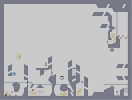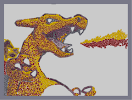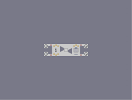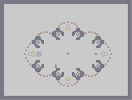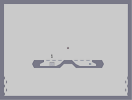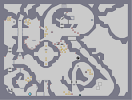Howdy Charizard A little brother No maps with unread comments Teleporters? Going Way to Fast

### This is awesome

but how did you edit already rated maps?
well it didn't make it to 100, but it's got my vote for the dronies ;]

### youd think

this to be a ded to jimi hendrix..

my vote..

### thats

a good nart for example

### probably

who it is a ded too turned away a few raters... plus espada posted his 2nd n-art of the palemoon/harry/nuke series while this was still at the top, so that replaced this...
I'm shocked that this didn't make it to 100 o_O way more accurate then your newest n-art, and cooler (imo)
fuckin god! its amazing! 75/5

### Woot, 40th rate.

Take a 5 'n. ;D GJ.

### at thepegasus

This is Why [nmaps.net]

Thanks everyone else =)

### liek OhMAIGAWd

i love jimmeh Hendrix!
sweet stuff.

### why

is this not first one on maps?

### better than the

last big nart!!!
just amazing :] 5

### /agree with Techno

although the beard is really nice

am i rite

fifty-eight

### I'm agreeing with AMLT on this one

It's really not that good.

Now espada's n-art. Let that live as an example of originality.

### O FUCK YES

THIS IS THE SHIT THIS IS THE SHIT THANK YOU
this r better than the avatar

its fine =]

### hey

I wasn't referring to execution, as this looks quite realistic. I was just citing evidence for amlt and rule's originality argument.

^^

### lol

This time it's Hendrix though. And it's a ded to me! BE HAPPY!!!!!!!!!

### Here we go again.

._.
pokmemon

thats even better than pokemon

its like waaay more extreme :3

### I did way better than that object n-art of

hendrix (the second one) and you cant really compare an object n-art like this with a tileset n-art =P

at least its not another pokmemon XD

### .

lol, totally agree with furry (again).

### hehe

this is Sooo [nmaps.net] Original! [nmaps.net]
well you guys fucked that up :/

no cookies for either of you D:
lol, totally agree with iban

### .

lol, totally agree with furry
lol, totally agree with karma

### very amassing

also fived to spite everyone
Oh noez. Not again.

WOOOOOOOOO!

### 5 outta 5

If you free handed this, then awesome!!!!

If you did use a program, then shame on you...